Note: The other languages of the website are Google-translated. Back to English

## 如何基于Excel中的单元格值将整行移动到另一张工作表？

#### 使用VBA代码根据单元格值将整行移动到另一张工作表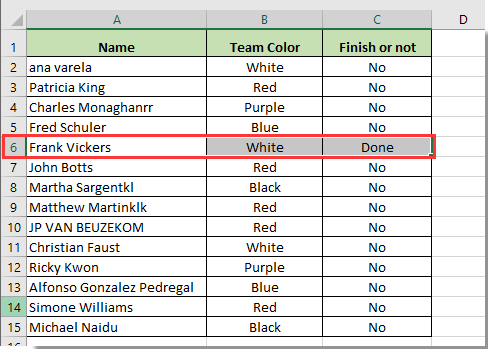1。 按 其他+ F11 同时打开 Microsoft Visual Basic应用程序 窗口。

2.在“ Microsoft Visual Basic for Applications”窗口中，单击“ 插页 > 模块。 然后将下面的VBA代码复制并粘贴到窗口中。

VBA code 1: Move entire row to another sheet based on cell value

``````Sub Cheezy()
'Updated by Kutools for Excel 2017/8/28
Dim xRg As Range
Dim xCell As Range
Dim I As Long
Dim J As Long
Dim K As Long
I = Worksheets("Sheet1").UsedRange.Rows.Count
J = Worksheets("Sheet2").UsedRange.Rows.Count
If J = 1 Then
If Application.WorksheetFunction.CountA(Worksheets("Sheet2").UsedRange) = 0 Then J = 0
End If
Set xRg = Worksheets("Sheet1").Range("C1:C" & I)
On Error Resume Next
Application.ScreenUpdating = False
For K = 1 To xRg.Count
If CStr(xRg(K).Value) = "Done" Then
xRg(K).EntireRow.Copy Destination:=Worksheets("Sheet2").Range("A" & J + 1)
xRg(K).EntireRow.Delete
If CStr(xRg(K).Value) = "Done" Then
K = K - 1
End If
J = J + 1
End If
Next
Application.ScreenUpdating = True
End Sub``````

3。 按 F5 键运行代码，然后将满足Sheet1中条件的行立即移至Sheet2。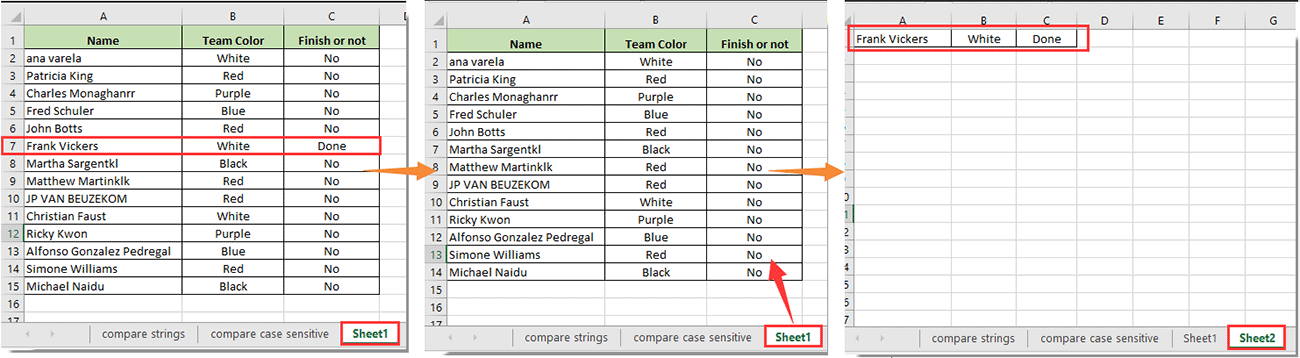VBA code 2: Copy entire row to another sheet based on cell value

``````Sub MoveRowBasedOnCellValue()
'Updated by Extendoffice 2017/11/10
Dim xRg As Range
Dim xCell As Range
Dim I As Long
Dim J As Long
Dim K As Long
I = Worksheets("Sheet1").UsedRange.Rows.Count
J = Worksheets("Sheet2").UsedRange.Rows.Count
If J = 1 Then
If Application.WorksheetFunction.CountA(Worksheets("Sheet2").UsedRange) = 0 Then J = 0
End If
Set xRg = Worksheets("Sheet1").Range("C1:C" & I)
On Error Resume Next
Application.ScreenUpdating = False
For K = 1 To xRg.Count
If CStr(xRg(K).Value) = "Done" Then
xRg(K).EntireRow.Copy Destination:=Worksheets("Sheet2").Range("A" & J + 1)
J = J + 1
End If
Next
Application.ScreenUpdating = True
End Sub``````

#### 使用Kutools for Excel根据单元格值将整行移动到另一张工作表

1.选择包含要作为行依据的单元格值的列列表，然后单击 库工具 > 选择 > 选择特定的单元格。 看截图：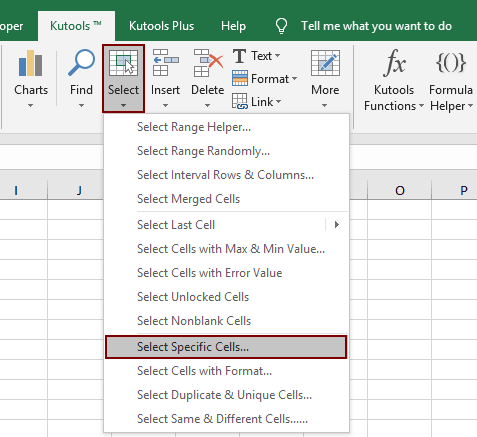2.在开幕 选择特定的单元格 对话框，选择 整行 ，在 选择类型 部分，选择 等于 ，在 特定类型 下拉列表，在文本框中输入单元格值，然后单击 OK 按钮。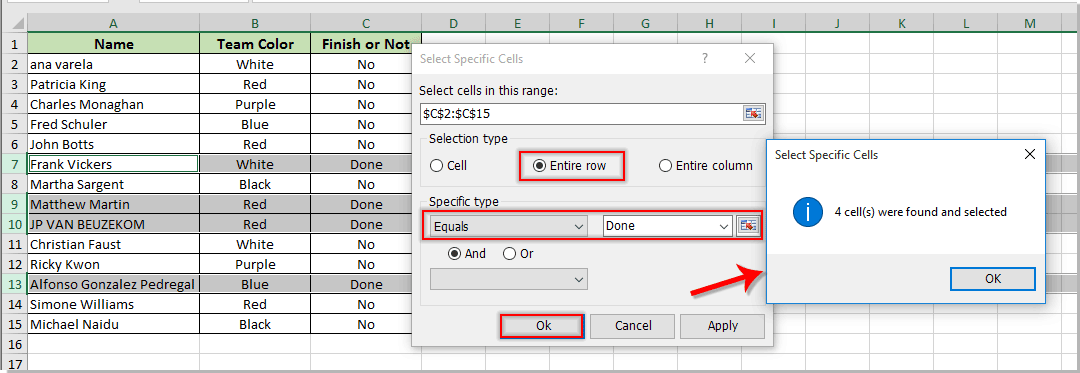3。 按 按Ctrl + C 键复制选定的行，然后将其粘贴到所需的目标工作表中。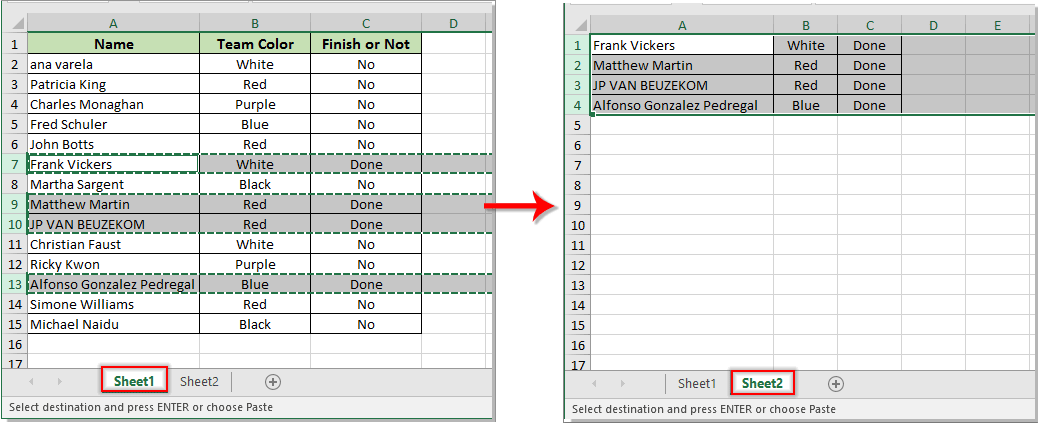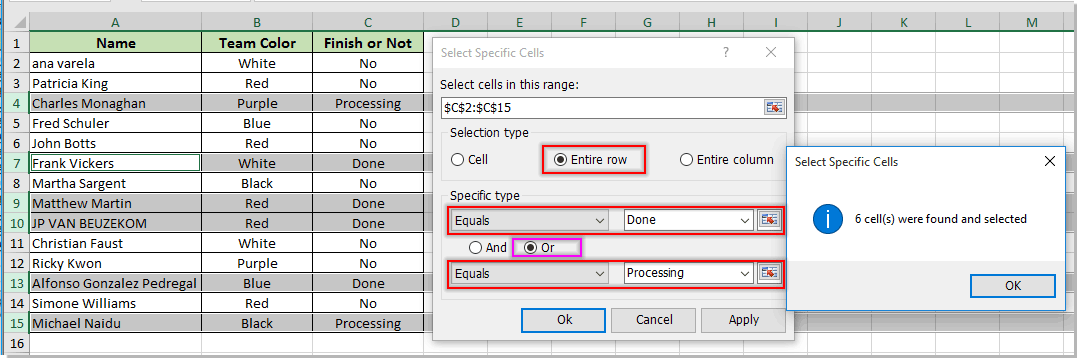如果您想免费试用（30-day) 这个实用程序， 请点击下载，然后按照上述步骤进行操作。

### 最佳办公效率工具

#### Kutools for Excel解决了您的大多数问题，并使您的生产率提高了80％

• 重用: 快速插入 复杂的公式，图表 以及您以前使用过的任何东西； 加密单元 带密码 创建邮件列表 并发送电子邮件...
• 超级公式栏 （轻松编辑多行文本和公式）； 阅读版式 （轻松读取和编辑大量单元格）； 粘贴到过滤范围...
• 合并单元格/行/列 不会丢失数据； 拆分单元格内容； 合并重复的行/列...防止细胞重复； 比较范围...
• 选择重复或唯一 行; 选择空白行 （所有单元格都是空的）； 超级查找和模糊查找 在许多工作簿中； 随机选择...
• 确切的副本 多个单元格，无需更改公式参考； 自动创建参考 到多张纸； 插入项目符号，复选框等...
• 提取文字，添加文本，按位置删除， 删除空间; 创建和打印分页小计； 在单元格内容和注释之间转换...
• 超级滤镜 （将过滤方案保存并应用于其他工作表）； 高级排序 按月/周/日，频率及更多； 特殊过滤器 用粗体，斜体...
• 结合工作簿和工作表; 根据关键列合并表； 将数据分割成多个工作表; 批量转换xls，xlsx和PDF...
• 超过300种强大功能. 支持 Office / Excel 2007-2021 和 365。支持所有语言。 在您的企业或组织中轻松部署。 完整功能 30 天免费试用。 60 天退款保证。#### Office选项卡为Office带来了选项卡式界面，使您的工作更加轻松

• 在Word，Excel，PowerPoint中启用选项卡式编辑和阅读，发布者，Access，Visio和Project。
• 在同一窗口的新选项卡中而不是在新窗口中打开并创建多个文档。
• 每天将您的工作效率提高50％，并减少数百次鼠标单击！Crystal 提供了有关如何执行此操作的信息 - 请查看此线程的第一页以查看她的回复。 它会自动将列中具有今天日期的行（在我的情况下为 L）移动到不同的工作表。

Sub Cheezy() Dim xRg As Range Dim xCell As Range Dim I As Long Dim J As Long I = Worksheets("Sheet1").UsedRange.Rows.Count J = Worksheets("Sheet2").UsedRange.Rows.Count If J = 1 Then If Application.WorksheetFunction.CountA(Worksheets("Sheet2").UsedRange) = 0 Then J = 0 End If Set xRg = Worksheets("Sheet1").Range("C1:C" & I) On Error Resume Next Application.ScreenUpdating = False For Each xCell In xRg If CStr(xCell.Value) = "Done" Then xCell.EntireRow.Copy Destination:=Worksheets("Sheet2").Range("A" & J + 1) xCell. EntireRow.Delete J = J + 1 End If Next Application.ScreenUpdating = True End Sub 如何添加第二个工作表以将行移动到 sheet2？

[quote]嗨，这很有帮助。 有没有办法在不将数据行移动到第二张工作表的情况下执行此操作，而是将其复制？ 那么数据会保留在两张纸上吗？通过麦迪[/quote] 有没有人解决这个问题

I = Worksheets("Sheet1").UsedRange.Rows.Count
J = Worksheets("Sheet2").UsedRange.Rows.Count

Application.ScreenUpdating = False

xRg(K).EntireRow.Copy Destination:=Worksheets("Sheet2").Range("A" & J + 1)
J = J + 1

Application.ScreenUpdating =真
END SUB

Application.ScreenUpdating = False

I = Worksheets("Sheet2").UsedRange.Rows.Count

xCell.EntireRow.Copy Worksheets("Sheet2").Range("A" & I + 1)

xCell.EntireRow.Delete

Application.ScreenUpdating =真

END SUB

Application.ScreenUpdating = False

I = Worksheets("Sheet2").UsedRange.Rows.Count

xCell.EntireRow.Copy Worksheets("Sheet2").Range("A" & I + 1)

xCell.EntireRow.Delete

Application.ScreenUpdating =真

END SUB

I = Worksheets("CURRENT OASIS OPPORTUNITIES").UsedRange.Rows.Count
J = Worksheets("ARCHIVED OASIS OPPORTUNITIES").UsedRange.Rows.Count

Application.ScreenUpdating = False

xCell.EntireRow.Copy Destination:=Worksheets("ARCHIVED OASIS OPPORTUNITIES").Range("A" & J + 1)
xCell.EntireRow.Delete
J = J + 1

END SUB

1.需要将VBA脚本放入ThisWorkbook代码窗口；
2. 您的工作簿需要保存为 Excel 启用宏的工作簿。

xLastRow = Worksheets("CURRENT OASIS OPPORTUNITIES").UsedRange.Rows.Count

J = Worksheets("ARCHIVED OASIS OPPORTUNITIES").UsedRange.Rows.Count

If xRg(I).Value > Date Then Exit Sub

xRg(I).EntireRow.Copy Destination:=Worksheets("ARCHIVED OASIS OPPORTUNITIES").Range("A" & J + 1)
xRg(I).EntireRow.删除
J = J + 1

END SUB

ABCDEFGHIJKL
# 类型预留征求修改 # 发布日期问题 客户交付地点 项目提案到期

1 SS SB 1234567 1 09/6/17 没有军队名称 Place Drive Tank 09/10/17

[模块 1 代码]

I = Worksheets("CURRENT OASIS OPPORTUNITIES").UsedRange.Rows.Count

J = Worksheets("ARCHIVED OASIS OPPORTUNITIES").UsedRange.Rows.Count

Application.ScreenUpdating = False

xCell.EntireRow.Copy Destination:=Worksheets("ARCHIVED OASIS OPPORTUNITIES").Range("A" & J + 1)
xCell.EntireRow.Delete

J = J + 1

Application.ScreenUpdating =真

END SUB

[表 1 代码]

Application.ScreenUpdating = False

I = Worksheets("ARCHIVED OASIS OPPORTUNITIES").UsedRange.Rows.Count

If Application.WorksheetFunction.CountA(Worksheets("ARCHIVED OASIS OPPORTUNITIES").UsedRange) = 0 Then I = 0 End If
xCell.EntireRow.Copy Worksheets("ARCHIVED OASIS OPPORTUNITIES").Range("A" & I + 1)
xCell.EntireRow.Delete

Application.ScreenUpdating =真
END SUB

'由 Kutools for Excel 更新 2017/8/28

I = Worksheets("PURCHASE FORCAST").UsedRange.Rows.Count
J = Worksheets("Purchase Archive").UsedRange.Rows.Count

Application.ScreenUpdating = False

xRg(K).EntireRow.Copy Destination:=Worksheets("Purchase Archive").Range("A" & J + 1)
xRg(K).EntireRow.删除

K = K - 1

J = J + 1

Application.ScreenUpdating =真
END SUB

``````Private Sub Worksheet_SelectionChange(ByVal Target As Range)
'Updated by Kutools for Excel 20220830
Dim xRg As Range
Dim xCell As Range
Dim I As Long
Dim J As Long
Dim K As Long
I = Worksheets("PURCHASE FORCAST").UsedRange.Rows.Count
J = Worksheets("Purchase Archive").UsedRange.Rows.Count
If J = 1 Then
If Application.WorksheetFunction.CountA(Worksheets("Purchase Archive").UsedRange) = 0 Then J = 0
End If
Set xRg = Worksheets("PURCHASE FORCAST").Range("H3:H" & I)
On Error Resume Next
Application.ScreenUpdating = False
For K = 1 To xRg.Count
If CStr(xRg(K).Value) = "Yes" Then
xRg(K).EntireRow.Copy Destination:=Worksheets("Purchase Archive").Range("A" & J + 1)
xRg(K).EntireRow.Delete
If CStr(xRg(K).Value) = "Yes" Then
K = K - 1
End If
J = J + 1
End If
Next
Application.ScreenUpdating = True
End Sub``````

https://www.extendoffice.com/product/kutools-for-excel/excel-select-specific-cells-rows.html

I = Worksheets("Sheet1").UsedRange.Rows.Count
J = Worksheets("Sheet2").UsedRange.Rows.Count

Application.ScreenUpdating = False
Application.CutCopyMode = False
xRgStatus(1).EntireRow.Copy
Worksheets("Sheet2").Range("A" & J + 1).PasteSpecial xlPasteAllUsingSourceTheme
J = J + 1

If (xRgDate(K).Value <> "") And (TypeName(xRgDate(K).Value) = "Date") 那么
xRgStatus(K).EntireRow.Copy
Worksheets("Sheet2").Range("A" & J + 1).PasteSpecial xlPasteAllUsingSourceTheme
J = J + 1

Application.CutCopyMode = True
Application.ScreenUpdating =真
END SUB

I = Worksheets("Sheet1").UsedRange.Rows.Count
J = Worksheets("Sheet2").UsedRange.Rows.Count

Application.ScreenUpdating = False

xRg(K).EntireRow.Copy Destination:=Worksheets("Sheet2").Range("A" & J + 1)
J = J + 1

Application.ScreenUpdating =真
END SUB

A1 = 蓝色
A5= 颜色
A6=（在此处转移“蓝色”）

## 关注我们

Microsoft和Office徽标是Microsoft Corporation在美国和/或其他国家的商标或注册商标。